Courses

# NEET Previous Year Questions (2014-20): Moving Charges and Magnetism Class 12 Notes | EduRev

## Class 12 : NEET Previous Year Questions (2014-20): Moving Charges and Magnetism Class 12 Notes | EduRev

The document NEET Previous Year Questions (2014-20): Moving Charges and Magnetism Class 12 Notes | EduRev is a part of the Class 12 Course Physics 28 Years Past year papers for NEET/AIPMT Class 12.
All you need of Class 12 at this link: Class 12

Q.1. A spherical conductor of radius 10 cm has a charge of 3.2 × 10-7 C distributed uniformly. what is the magnitude of electric field at a point 15 cm from the centre of the sphere?      (2020)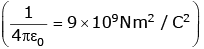Ans: D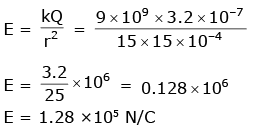Q.2. A long sol enoid of 50 cm length having 100 turns carries a current of 2.5 A. The magnetic field at the centre of the solenoid is : (μ0 = 4π × 10-7 Tm A-1)       (2020)
A: 6.28×10-5 T
B: 3.14×10-5 T
C: 6.28×10-4 T
D: 3.14×10-4 T
Ans:
C
Magnetic field for solenoid is given by
B = μ0 nI
n = N/ℓ
= 4π × 10-7 × 200 × 2.5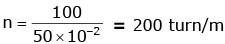= 4 × 3.14 × 10–7 × 500
= 2000 × 3.14 × 10–7
= 6.14 × 10–4 T

Q.3. A cylindrical conductor of radius R is carrying a constant current. The plot of the magnitude of the magnetic field. B with the distance d from the centre of the conductor, is correctly represented by the figure :      (2019)
A: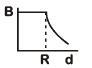B: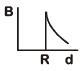C: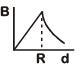D: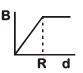Ans:
C
Solution: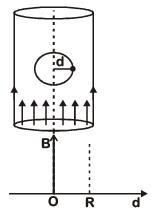Inside (d < R) Magnetic field inside conductor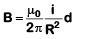or B = Kd   ...(i)
Straight line passing through origin
At surface (d = R)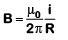...(ii)
Maximum at surface Outside (d > R)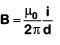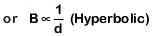Q.4. Ionized hydrogen atoms and α-particles with same momenta enters perpendicular to a constant magnetic field, B. The ratio of their radii of their paths rH : ra will be :     (2019)
A: 2 : 1
B: 1 : 2
C: 4 : 1
D: 1 : 4
Ans:
A
Solution: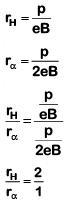Q.5. A 250-Turn rectangular coil of length 2.1 cm and width 1.25 cm carries a current of 85 µA and subjected to magnetic field of strength 0.85 T. Work done for rotating the coil by 180º against the torque is:-       (2017)
A: 4.55 µJ
B: 2.3 µJ
C: 1.15 µJ
D: 9.1 µJ
Ans:
D
Solution:

W = MB (cosθ1– cosθ2)
When it is rotated by angle 180º then
W = 2MB
W = 2 (NIA)B
= 2 x 250 x 85 x 10-6[1.25 x 2.1 x 10-4] x 85 x 10-2
= 9.1μJ

Q.6. An arrangement of three parallel straight wires placed perpendicular to plane of paper carrying same current 'I' along the same direction is shown in fig. Magnitude of force per unit length on the middle wire 'B' is given by :-      (2017)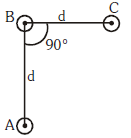A: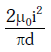B: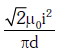C: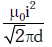D: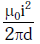Ans:
C
Solution: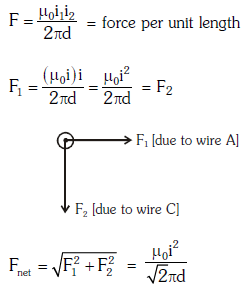Q.7. A long straight wire of radius a carries a steady current I. The current is uniformly distributed over its cross-section. The ratio of the magnetic fields B and B`, at a radial distances a/2 and 2a respectively, from the axis of the wire is:     (2016)
A: 4
B: 1/4
C: 1/2
D: 1
Ans:
D
Solution:

Consider two amperian loops of radius a/2 and 2a as shown in the diagram.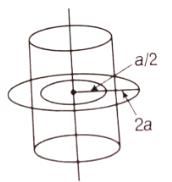Applying Ampere's circuital law for these loops, we get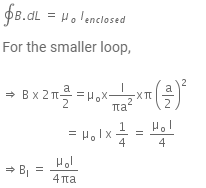B1 acts at a distance a/2 from the axis of the wire.
Similarly, for bigger amperian loop,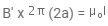Total current enclosed by amperian loop 2.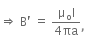at a distance 2a from the axis of the wire.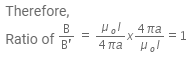Q.8. Two identical long conducting wires AOB and COD are placed at right angle to each other, with one above other such that ‘O’ is their common point for the two. The wires carry I1 and I2 currents, respectively. Point ‘P’ is lying at distance ‘d’ from ‘O’ along a direction perpendicular to the plane containing the wires. The magnetic field at the point ‘P’ will be :     (2014)
A: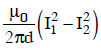B: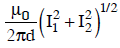C: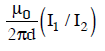D: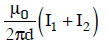Ans: B
Solution: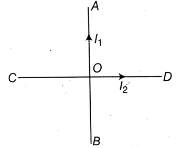The point P is lying at a distance d along the z-axis.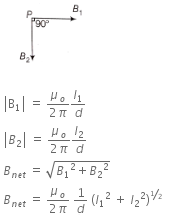Offer running on EduRev: Apply code STAYHOME200 to get INR 200 off on our premium plan EduRev Infinity!

14 docs|25 tests

,

,

,

,

,

,

,

,

,

,

,

,

,

,

,

,

,

,

,

,

,

;